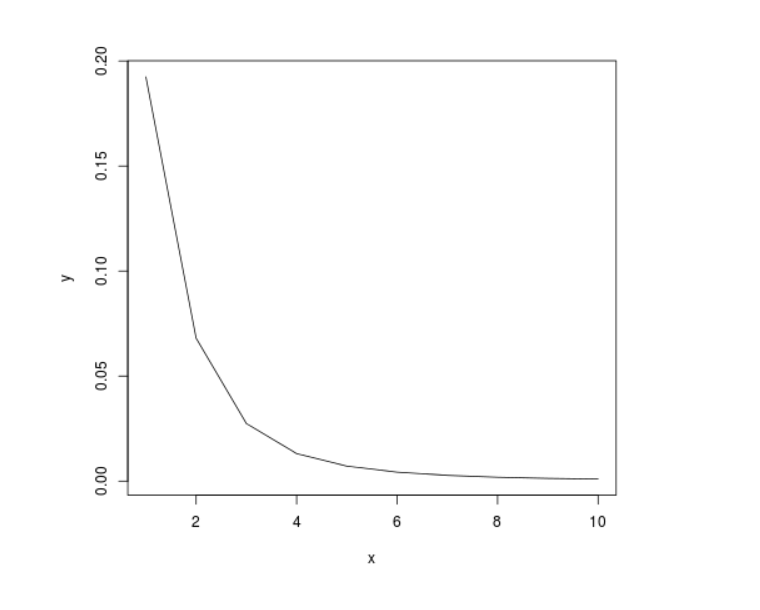Skip to content
Related Articles
Perform Probability Density Analysis on t-Distribution in R Programming – dt() Function
• Last Updated : 19 Jun, 2020

`dt()` function in R Language is used to return the probability density analysis on the Student t-distribution with random variable x and degree of freedom df.

Syntax: dt(x, df)

Parameters:
x: Random Variable
df: Degree of Freedom

Example 1:

 `# R Program to perform``# Probability Density Analysis`` ` `# Calling dt() Function``dt(``0``, ``10``)``dt(``1``, ``15``)``dt(``3``, ``40``)`

Output:

``` 0.3891084
 0.2341248
 0.006185947
```

Example 2:

 `# R Program to perform``# Probability Density Analysis`` ` `# Creating a vector``x <``-` `seq(``1``, ``10``, by ``=` `1``)`` ` `# Calling dt() Function``y <``-` `dt(x, ``2``)`` ` `# Plotting probability distribution graph``plot(x, y, ``type` `=``"l"``)`

Output:My Personal Notes arrow_drop_up